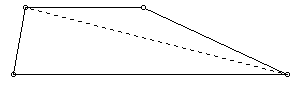# Triangles Inside a TrapezoidA diagonal of a trapezoid divides it into two triangles. Describe the trapezoid(s) formed when the triangles have equal areas. Explain why these types of trapezoids are formed.Extension
Use the formula for a triangle to show that the area of a trapezoid is one-half the product of the height and sum of the bases.